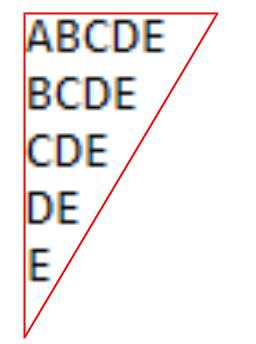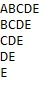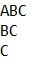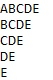# Program for triangular patterns of alphabets in C

Given a number n, the task is to print the triangular patterns of alphabets of the length n. First print the n characters then decrement one from the beginning in each line.

The triangular pattern of alphabet will be like in the given figure below −Input − n = 5

OutputInput − n = 3

Output## Approach used below is as follows to solve the problem

• Take input n and loop i from 1 to n.

• For every i iterate j from i to n for every j print a character subtract 1 and add the value of j to ‘A’ .

## Algorithm

Start
In function int pattern( int n)
Step 1→ Declare int i, j
Step 2→ Loop For i = 1 and i < n and i++
Loop For j = i and j <= n and j++
Print 'A' - 1 + j
Print new line
In function int main()
Step 1→ Declare and initialize n = 5
Step 2→ call pattern(n)
Stop

## Example

Live Demo

#include <stdio.h>
int pattern( int n){
int i, j;
for (i = 1; i <= n; i++) {
for (j = i; j <= n; j++) {
printf("%c", 'A' - 1 + j);
}
printf("");
}
return 0;
}
int main(){
int n = 5;
pattern(n);
return 0;
}

## Output

If run the above code it will generate the following output −